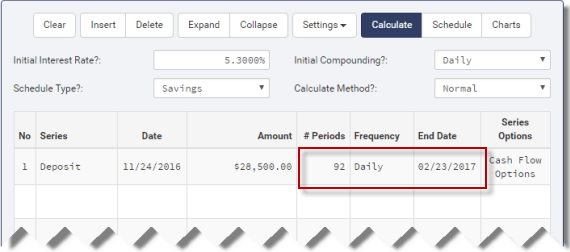# Time Value of Money TutorialsTVM Calculations

## How to Calculate Return on an Investment for "X" DaysTutorial 24

Sometimes it is necessary to know the return on an investment for a specific number of days.

For example, if you invest in money market funds or CDs, the term can be measured in days. Or even if you trade long term bonds you may need to know the accrued interest since the last coupon date. (Accrued interest is the return on the investment.) The Ultimate Financial Calculator can calculate the return for a specified number of days. This tutorial will illustrate how it is done.

For this example, we are assuming you need to know the return for a given number of days, but you don't know the future value date. Meaning, you want to know the interest earned for 92 days, but you don't know what date is 92 days from the initial investment date. Step 4 in this tutorial illustrates how to calculate the final (FV) date. If you already know the FV date, then, skip 4d and 4e and enter 1 for frequency.

This example applies to our online Ultimate Financial Calculator. The C-Value! program for Windows works in a similar way and has a few more features including the ability to save your work.

All users should work through the first tutorial to understand basic concepts about the calculator.

To calculate the return for a specific number of days, follow these steps:

1. Set "Schedule Type" to "Savings"
• Or click the [Clear] button to clear any previous entries.
• The top two rows of the grid will not be empty
• Delete the 2nd row by selecting it and clicking on the [Delete] button
2. Set "Rounding" to "Open balance — no adjustment" by clicking on {Settings} {Rounding Options}
3. Set initial values for an investment cash flow in the header area.
• Both interest rate and compounding can be changed on later dates (see tutorial #4)
1. For "Initial Interest Rate", enter 5.3 for 5.3%
2. Set "Initial Compounding" to "Daily".
3. For "Calculate Method" select "Normal".
1. Click on row one of the cash flow grid
1. Set the "Series" to "Deposit"
2. Set the "Date" to 11/24/2016  (mm/dd/yyyy)
3. Set the "Amount" to 28,500.00
4. Set the "# Periods" to 92
5. Set the "Frequency" to "Daily".
• Find the date 92 days from the start date — February 23, 2017
• This is automatically calculatedCalculate date "92" days from investment date — February 23, 2017
1. Create a "Withdrawal" series in row two — which we'll come back to in the next step
• Assume we are calculating the accrued interest for a bond
• Bonds typically compound interest semiannually
1. Reset "Initial Compounding" to "Semiannually"
2. Reset "# Periods" in row one to 1
1. Set the details of the "Withdrawal" series in row 2
1. The "Date" should already be set to 02/24/2017
• Step 4 calculates the last of the 92 days as February 23, 2017. But we need 92 twenty-four hour periods which ends on February 24. Think of it this way. Two days would be November 24th and November 25th but you can't make a deposit on November 24th and withdrawal it on November 25th and expect 2 days of interest. To earn interest for two days you have to withdrawal the funds on November 26th. Same concept applies here.
2. Set the "Amount" to "Unknown" by typing "U"
3. Set the "# Periods" to 1
• We are now ready to calculate the unknown value. Your screen should look like this: